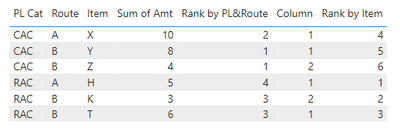cancel
Showing results for
Did you mean:Helper IV

## Rankx display

Hi

I have table 1 is power BI. I wanna create a matrix like table 2 with below logic:

- Rank by PL & Route: Ranking the Amt by PL Cat & Route

- Rank by Item: Ranking the Amt by Item only

So, How to make a measure to do it?2 ACCEPTED SOLUTIONSSuper User

@ngocnguyen , Try ranks like

ranxk(allselected(Table[Item]), calculate(sum(Table[Amount]), allexcept(Table, Table[Item])),,desc,dense)

ranxk(Summarize(allselected(Table),Table[PL cat],Table[item] ), calculate(sum(Table[Amount]), allexcept(Table, Table[PL cat], Table[item])),,desc,dense)

Power BI Rank Across dimension tables: https://youtu.be/X59qp5gfQoACommunity Support

Hi @ngocnguyen ,

According to your description, here's my solution. Create calculated columns.

``````Rank by PL&Route =
RANKX (
'Table 1',
SUMX (
FILTER (
ALL ( 'Table 1' ),
'Table 1'[PL Cat] = EARLIER ( 'Table 1'[PL Cat] )
&& 'Table 1'[Route] = EARLIER ( 'Table 1'[Route] )
),
'Table 1'[Amt]
),
,
DESC,
DENSE
)
``````

For the second "Rank by Item", your requirement is Ranking the Amt by Item only, but the result in Table2 is not ranking by item. So I provide two formula here.

If you really want to rank by Item:

``````Rank by Item =
RANKX ( 'Table 1', 'Table 1'[Item],, ASC )
``````

If you want to get the provided result:

``````Column =
RANKX (
FILTER (
'Table 1',
'Table 1'[Route] = EARLIER ( 'Table 1'[Route] )
&& 'Table 1'[PL Cat] = EARLIER ( 'Table 1'[PL Cat] )
),
'Table 1'[Amt]
)
``````

Result:I attach my sample below for your reference.

Best Regards,
Community Support Team _ kalyj

If this post helps, then please consider Accept it as the solution to help the other members find it more quickly.

2 REPLIES 2Community Support

Hi @ngocnguyen ,

According to your description, here's my solution. Create calculated columns.

``````Rank by PL&Route =
RANKX (
'Table 1',
SUMX (
FILTER (
ALL ( 'Table 1' ),
'Table 1'[PL Cat] = EARLIER ( 'Table 1'[PL Cat] )
&& 'Table 1'[Route] = EARLIER ( 'Table 1'[Route] )
),
'Table 1'[Amt]
),
,
DESC,
DENSE
)
``````

For the second "Rank by Item", your requirement is Ranking the Amt by Item only, but the result in Table2 is not ranking by item. So I provide two formula here.

If you really want to rank by Item:

``````Rank by Item =
RANKX ( 'Table 1', 'Table 1'[Item],, ASC )
``````

If you want to get the provided result:

``````Column =
RANKX (
FILTER (
'Table 1',
'Table 1'[Route] = EARLIER ( 'Table 1'[Route] )
&& 'Table 1'[PL Cat] = EARLIER ( 'Table 1'[PL Cat] )
),
'Table 1'[Amt]
)
``````

Result:I attach my sample below for your reference.

Best Regards,
Community Support Team _ kalyj

If this post helps, then please consider Accept it as the solution to help the other members find it more quickly.Super User

@ngocnguyen , Try ranks like

ranxk(allselected(Table[Item]), calculate(sum(Table[Amount]), allexcept(Table, Table[Item])),,desc,dense)

ranxk(Summarize(allselected(Table),Table[PL cat],Table[item] ), calculate(sum(Table[Amount]), allexcept(Table, Table[PL cat], Table[item])),,desc,dense)

Power BI Rank Across dimension tables: https://youtu.be/X59qp5gfQoAAnnouncements#### Exclusive opportunity for Women!

Join us for a free, hands-on Microsoft workshop led by women trainers for women where you will learn how to build a Dashboard in a Day!#### Power Platform Conference-Power BI and Fabric Sessions

Join us Oct 1 - 6 in Las Vegas for the Microsoft Power Platform Conference.Top Solution Authors
Top Kudoed Authors
Users online (2,052)